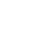# pybind11的基本入门操作--使用示例pybind11是一个只包含头文件的轻量级库，可以通过它实现C/C++接口的调用，相比于boost.python, swig, ctypes等，pybind11的优点是API比较简单且对C++ 11支持很好。下面介绍pybind11的基本入门操作。

（1）源码下载

``git clone https://github.com/pybind/pybind11.git``

（2）创建C++函数与python的绑定关系

``````#include
namespace py = pybind11;
int add(int a, int b) {
return a + b;
}
PYBIND11_MODULE(example, m) {
}``````

（3）编译

pybind11是一个仅有头文件的库，所以不需要链接其它的库，在Linux环境下，可以用以下的命令进行编译：

``g++ -O3 -Wall -shared -std=c++11 -fPIC `python3 -m pybind11 --includes` example.cpp -o example.so``

``-I/software/conda_envs/py35/include/python3.5m -I/software/pybind11/include``

``````>>> import example as e
3``````

（4）设置参数默认值

C++可以为函数的参数设置默认值，但是pybind11目前并不会自动提取这些默认值，可以通过pybind11::arg()来指定：

``````m.def("add", &add, "A function which adds two numbers"，
py::arg("a") = 1, py::arg("b") = 2);``````

``m.def("add", &add, "a"_a=1, "b"_a=2);``

（5）导出变量

``````PYBIND11_MODULE(example, m) {
m.attr("age") = 42;
py::object name = py::cast("Oreo");
m.attr("name") = name;
}``````

``````>>> import example as e
>>> print(e.age)
42
>>> print(e.name)
'Oreo'``````

《pybind11的基本入门操作--使用示例》